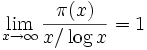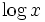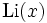# Prime number theorem

## Statement

### Statement in terms of the asymptotic distribution in ratio terms

This states that:whereis the prime-counting function: the number of primes less than or equal to, andis the natural logarithm, i.e., the logarithm to base.

### Statement in terms of the logarithmic integral

This is a stronger formulation, which states that:.

Here,denotes the logarithmic integral.